# Excel Data Validation: custom formulas and rules

The tutorial shows how to make custom Data Validation rules in Excel. You will find a few examples of Excel data validation formulas to allow only numbers or text values in specific cells, or only text beginning with specific characters, permit unique data preventing duplicates, and more.

In yesterday's tutorial we started to look at Excel Data Validation - what its purpose is, how it works, and how to use built-in rules to validate data in your worksheets. Today, we are going to take a step further and talk about the nitty-gritty aspects of custom data validation in Excel as well as experiment with a handful of different validation formulas.

## How to create custom data validation with formula

Microsoft Excel has several built-in data validation rules for numbers, dates and text, but they cover only the most basic scenarios. If you want to validate cells with your own criteria, create a custom validation rule based on a formula. Here's how:

1. Select one or more cells to validate.
2. Open the Data Validation dialog box. For this, click the Data Validation button on the Data tab, in the Data Tools group or press the key sequence Alt > D > L (each key is to be pressed separately).
3. On the Settings tab of the Data Validation dialog window, select Custom in the Allow box, and enter your data validation formula in the Formula box.
4. Click OK.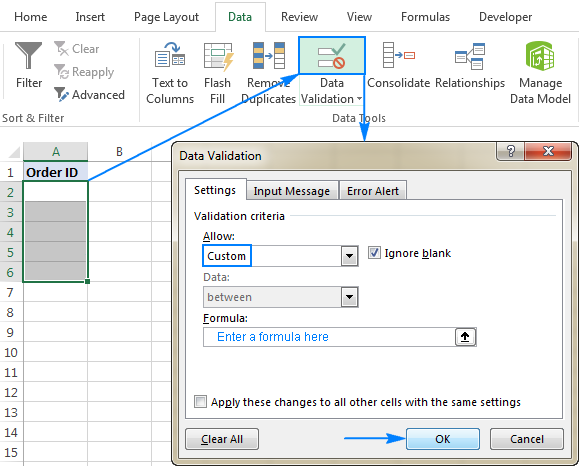Optionally, you can add a custom input message and Error alert that will show up when the user selects the validated cell or enters invalid data, respectively.

Below you will find a few examples of custom validation rules for different data types.

Note. All Excel data validation rules, built-in and custom, verify only new data that is typed in a cell after creating the rule. Copied data is not validated, nor is the data input in the cell before making the rule. To pin down existing entries that do not meet your data validation criteria, use the Circle Invalid Data feature as shown in How to find invalid data in Excel.

## Excel data validation to allow numbers only

Surprisingly, none of the inbuilt Excel data validation rules cater for a very typical situation when you need to restrict users to entering only numbers in specific cells. But this can be easily done with a custom data validation formula based on the ISNUMBER function, like this one:

`=ISNUMBER(C2)`

Where C2 is the topmost cell of the range you want to validate.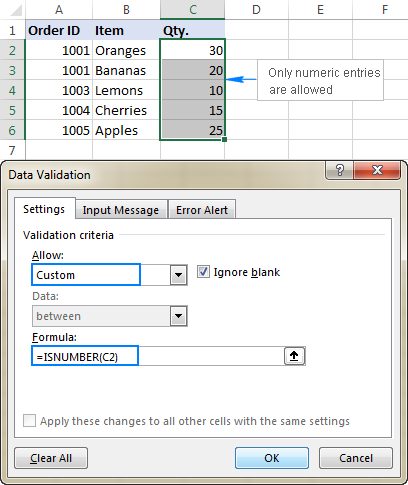Note. The ISNUMBER function allows any numeric values in validated cells, including integers, decimals, fractions as well as dates and times, which are also numbers in terms of Excel.

## Excel data validation to allow text only

If you are looking for the opposite - to allow only text entries in given range of cells, then build a custom rule with the ISTEXT function, for example:

`=ISTEXT(D2)`

Where D2 is the uppermost cell of the selected range.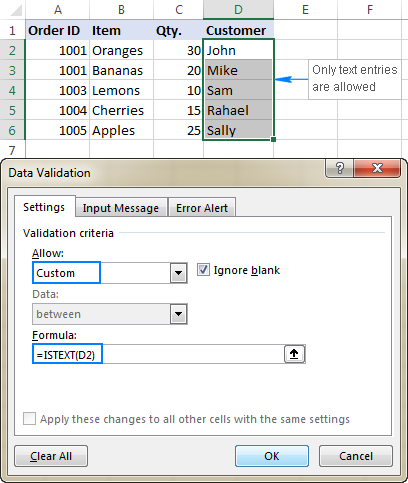## Allow text beginning with specific character(s)

If all values in a certain range should begin with a particular character or substring, then do Excel data validation based on the COUNTIF function with a wildcard character:

COUNTIF(cell,"text*")

For example, to ensure that all order id's in column A begin with the "AA-", "aa-", "Aa-", or "aA-" prefix (case-insensitive), define a custom rule with this data validation formula:

`=COUNTIF(A2,"aa-*")`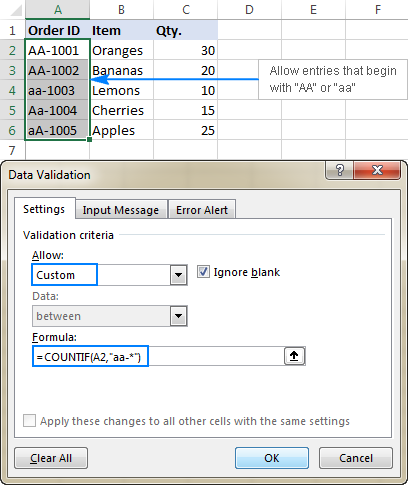#### Validation formula with the OR logic (multiple criteria)

In case there are 2 or more valid prefixes, add up several COUNTIF functions, so that your Excel data validation rule works with the OR logic:

`=COUNTIF(A2,"aa-*")+COUNTIF(A2,"bb-*")`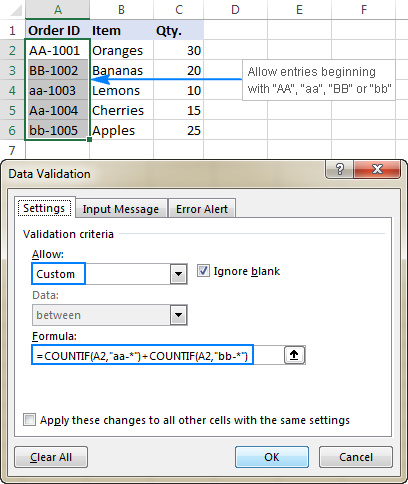#### Case-sensitive validation formula

If the character case matters, then use EXACT in combination with the LEFT function to create a case-sensitive validation formula for entries beginning with specific text:

EXACT(LEFT(cell, number_of_chars), text)

For instance, to allow only those order ids that begin with "AA-" (neither "aa-" nor "Aa-" is allowed), use this formula:

`=EXACT(LEFT(A2,3),"AA-")`

In the above formula, the LEFT function extracts the first 3 characters from cell A2, and EXACT performs a case-sensitive comparison with the hard-coded substring ("AA-" in this example). If the two substrings match exactly, the formula returns TRUE and the validation passes; otherwise FALSE is returned and the validation fails.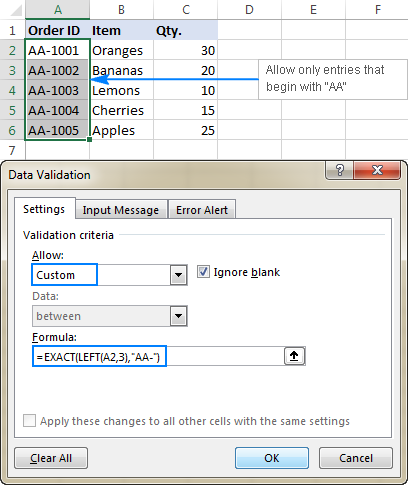## Allow entries containing certain text

To allow entries that contain specific text anywhere in a cell (in the beginning, middle, or end), use the ISNUMBER function in combination with either FIND or SEARCH depending on whether you want case-sensitive or case-insensitive match:

• Case-insensitive validation:

ISNUMBER(SEARCH(text, cell))
• Case-sensitive validation:

ISNUMBER(FIND(text, cell))

On our sample data set, to permit only entries containing the text "AA" in cells A2:A6, use one of these formulas:

Case-insensitive:

`=ISNUMBER(SEARCH("AA", A2))`

Case-sensitive:

`=ISNUMBER(FIND("AA", A2))`

The formulas work with the following logic:

You search the substring "AA" in cell A2 using FIND or SEARCH, and both return a position of the first character in the substring. If the text is not found, an error is returned. For any numeric value returned as the result of search, the ISNUMBER function yields TRUE, and data validation is successful. In case of an error, ISNUMBER returns FALSE, and the entry won't be allowed in a cell.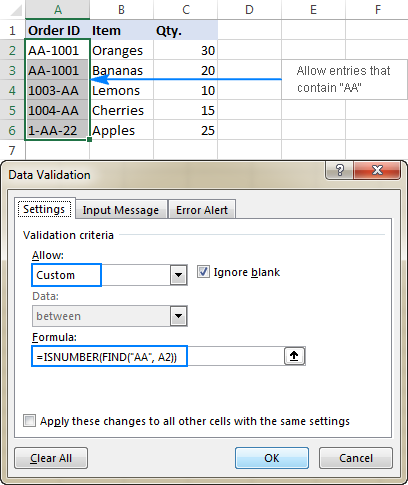## Data validation to allow only unique entries and disallow duplicates

In situations when a certain column or a range of cell should not contain any duplicates, configure a custom data validation rule to allow only unique entries. For this, we are going to use the classic COUNTIF formula to identify duplicates:

=COUNTIF(range, topmost_cell)<=1

For example, to make sure that only unique order ids are input in cells A2 to A6, create a custom rule with this data validation formula:

`=COUNTIF(\$A\$2:\$A\$6, A2)<=1`

When a unique value is entered, the formula returns TRUE and the validation succeeds. If the same value already exists in the specified range (count greater than 1), COUNTIF returns FALSE and the input fails validation.

Please pay attention that we lock the range with absolute cell references (A\$2:\$A\$6) and use a relative reference for the top cell (A2) to get the formula to adjust properly for each cell in the validated range.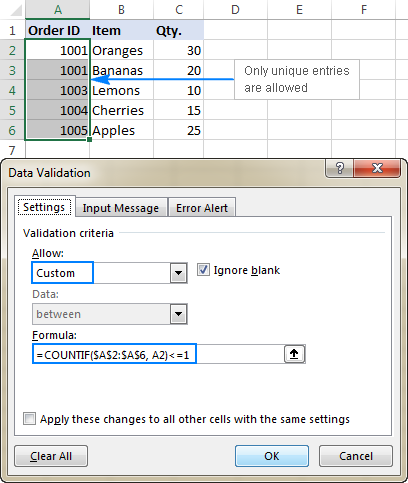Note. This data validation formulas is case-insensitive, it does not distinguish uppercase and lowercase text.

## Validation formulas for dates and times

Inbuilt date validation provides quite a lot of predefined criteria to restrict users to entering only dates between the two dates you specify, greater than, less than, or equal to a given date.

If you want more control over data validation in your worksheets, you can replicate the inbuilt functionality with a custom rule or write your own formula that goes beyond the built-in capabilities of Excel data validation.

### Allow dates between two dates

To limit the entry to a date within a specified range, you can use either the predefined Date rule with the "between" criteria or make a custom validation rule with this generic formula:

AND(cell>=start_date), cell<=end_date)

Where:

• cell is the topmost cell in the validated range, and
• start and end dates are valid dates supplied via the DATE function or references to cells containing the dates.

For example, to allow only dates in the month of July of the year 2017, use the following formula:

`=AND(C2>=DATE(2017,7,1),C2<=DATE(2017,7,31))`

Or, enter the start date and end date in some cells (F1 and F2 in this example), and reference those cells in your formula:

`=AND(C2>=\$F\$1, C2<=\$F\$2)`

Please notice that the boundary dates are locked with absolute cell references.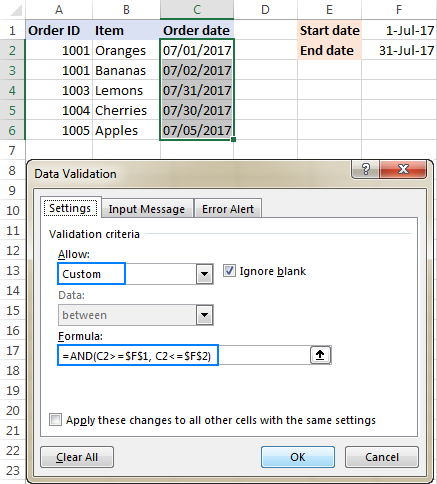### Allow weekdays or weekends only

To restrict a user to entering only weekdays or weekends, configure a custom validation rule based on the WEEKDAY function.

With the return_type argument set to 2, WEEKDAY returns an integer ranging from 1 (Monday) to 7 (Sunday). So, for weekdays (Mon to Fri) the result of the formula should be less than 6, and for weekends (Sat and Sun) greater than 5.

Allow only workdays:

WEEKDAY(cell,2)<6

Allow only weekends:

WEEKDAY(cell,2)>5

For example, to allow entering only workdays in cells C2:C6, use this formula:

`=WEEKDAY(C2,2)<6`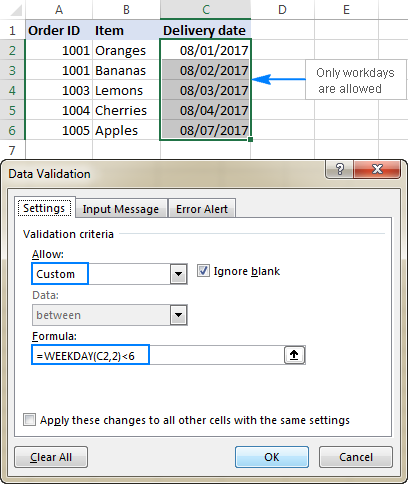### Validate dates based on today's date

In many situations, you may want to use today's date as the start date of the allowed date range. To get the current date, use the TODAY function, and then add the desired number of days to it to compute the end date.

For example, to limit the data entry to 6 days from now (7 days including today), we are going to use the built-in Date rule with the formula-based criteria:

1. Select Date in the Allow
2. Select between in the Data
3. In the Start date box, enter `=TODAY()`
4. In the End date box, enter `=TODAY() + 6`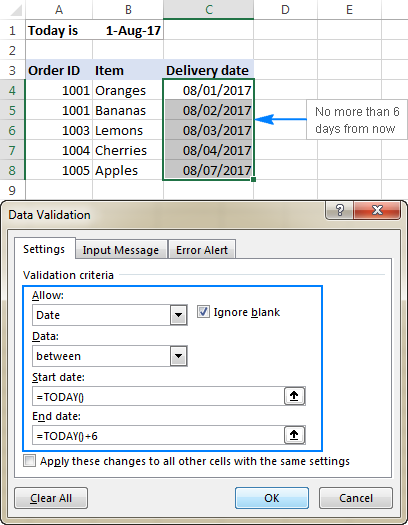In a similar manner, you can restrict users to entering dates before or after today's date. For this, select either less than or greater than in the Data box, and then enter `=TODAY()` in the End date or Start date box, respectively.

### Validate times based on current time

To validate data based on the current time, use the predefined Time rule with your own data validation formula:

1. In the Allow box, select Time.
2. In the Data box, pick either less than to allow only times before the current time, or greater than to allow times after the current time.
3. In the End time or Start time box (depending on which criteria you selected on the previous step), enter one of the following formulas:
• To validate dates and times based on the current date and time:
`=NOW()`
• To validate times based on the current time:
`=TIME( HOUR(NOW()), MINUTE(NOW()), SECOND(NOW()))`

The screenshot below shows a rule that allows only times greater than the current time: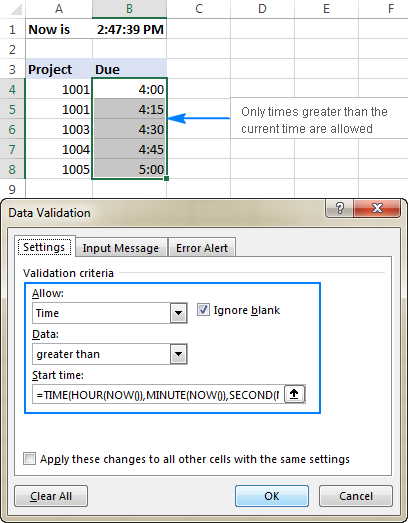## Custom Excel data validation rule not working

If your formula-based data validation rule does not work as expected, there are 3 main points to check:

• Data validation formula is correct
• Validation formula does not refer to an empty cell
• Appropriate cell references are used

### Check the correctness of your Excel data validation formula

For starters, copy your validation formula into some cell to make sure it does not return an error such as #N/A, #VALUE or #DIV/0!.

If you are creating a custom rule, the formula should return the logical values of TRUE and FALSE or the values of 1 and 0 equating to them, respectively.

If you use a formula-based criteria in a built-in rule (like we did to validate times based on the current time), it can also return another numeric value.

### Excel data validation formula should not refer to an empty cell

In many situations, if you select the Ignore blank box when defining the rule (usually selected by default) and one or more cells referenced in your formula is blank, any value will be allowed in the validated cell.

Here is an example in the simplest form: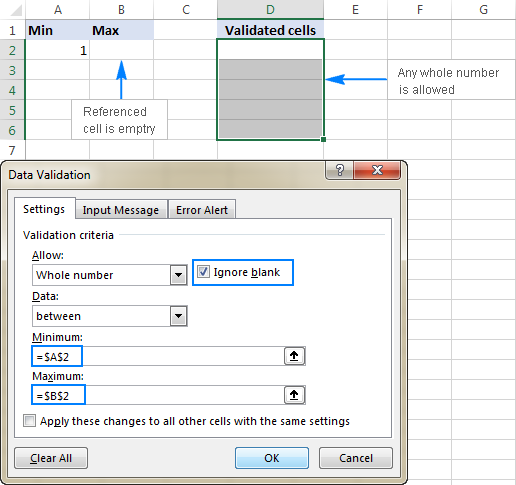### Absolute and relative cell references in data validation formulas

When setting up a formula-based Excel validation rule, please keep in mind that all cell references in your formula are relative to the upper left cell in the selected range.

If you are creating a rule for more than one cell and your validation criteria are dependent on specific cells, be sure to use absolute cell references (with the \$ sign like \$A\$1), otherwise your rule will work correctly only for the first cell. To better illustrate the point, please consider the following example.

Supposing, you want to restrict data entry in cells D2 to D5 to whole numbers between 1 (minimum value) and the result of dividing A2 by B2. So, you calculate the maximum value with this simple formula `=A2/B2`, like shown in the screenshot below: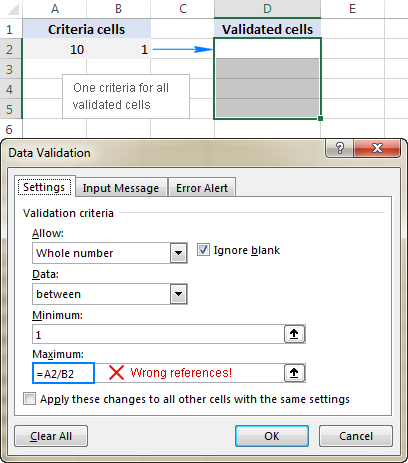The problem is this seemingly correct formula won't work for cells D3 to D5 because relative references change based on a relative position of rows and columns. Thus, for cell D3 the formula will change to `=A3/B3`, and for D4 it will become `=A4/B4`, doing data validation all wrong!

To fix the formula, just type "\$" before the column and row references to lock them: `=\$A\$2/\$B\$2`. Or, press F4 to toggle between different reference types.

In situations when you want to validate each cell based on its own criteria, use relative cell references without \$ sign to get the formula to adjust for each row or/and column: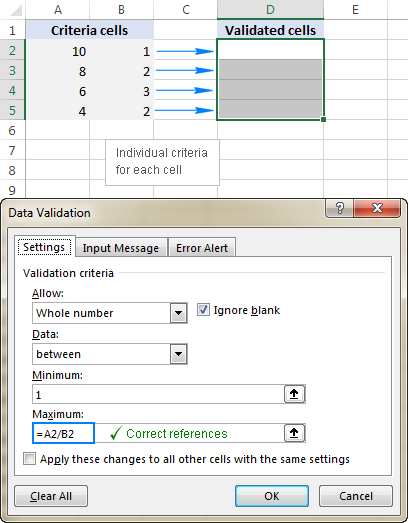As you see, there is no "absolute truth", the same formula could be right or wrong depending on situation and your particular task.

This is how to use data validation in Excel with your own formulas. T gain more understanding, feel free to download our sample workbook below and examine the rule settings. I thank you for reading and hope to see you on our blog next week!

Excel Data Validation examples (.xlsx file)

## You may also be interested in

1. Hello Mr. Alexander, and I hope you have a great day ahead of you. I actually need your dire help regarding the data validation formula.

Here is my problem, now that I have a formula that only allows 1 number not greater or lesser than 1 (negative numbers) but, however, I have tried to add another "1" on older cell, for example, I have added 1 on C17 and when I want to add 1 before C17 which is C16 it shows an error message, which is that what I want to implement when I add greater than 1 or some negative numbers.

Here is my current formula: "=AND(SUM(C5:C17)=1)" and I want to solve this particular issue for my monitoring.

• I add 1 on C17 there's no error message but, when I add 1 on C16 and other cells before it, it gave me an error message.

Am I doing it wrong or can I have a proper formula about it?

• Hi! I don't really understand your problem. Your formula allows only one number 1 to be written into the range C5:C17 and only in one cell. What do you want to do?

• Hello sir, sorry for the unclear statement.

Actually, I have this monitoring Excel file for a specific department as requested by the department's manager. And this monitoring can be used to overseer the stocks of those inks. What we ask this department staff is to request only 1 ink after their current ink is empty so we can properly handle the ink stocks and proceed to encode the date released. They cannot request more than one ink.

So the kind of Data Validation I implement is if the value exceeds to greater than 1, an error message will appear stating, "invalid request". Again, sorry for not explaining clearly earlier.

2. Hi,
I have created a formula that returns true and false when desired. However, when applied in the data validation, it only partially works.
I have a cell E4 in which the person inputs a list. The list format is string, string, string. For instance, E4 = Group 2, Team B, XZZ
Then, in E5, the person must type strings that belong in the listed strings in E4. Same list format. For instance, E5 = XZZ, Group 2
I want to ensure that indeed the strings used in E5 are strings that exist in E4, and case sensitive.
To do so, I came up with the following formula:
=EXACT(TEXTJOIN(", ";TRUE;IFNA(VLOOKUP(TEXTSPLIT(\$E5;;", ");TEXTSPLIT(\$E\$4;;", ");1;FALSE);"#pb#"));\$E5)
The formula returns TRUE, because indeed E5 (XZZ, Group 2) is made of strings that belong in E4 ( Group 2, Team B, XZZ) and respect the list format (string, string, string).

However, when I use this formula for data validation, it returns an error. The input in E5 is invalid according to the data validation.
If I have only 1 string in E5, for instance E5= XZZ, then the data validation does NOT return an error.

I don't understand why the formula works in the sheet but not in data validation.

• Hi! If E5= XZZ, then your formula returns TRUE. Therefore, the data validation does not return an error. This is consistent with the conditions you described. "XZZ" is present in "Group 2, Team B, XZZ".

3. Hi, I want to create a validation where each cell cannot be greater than a certain number (say 10) and the sum of certain numbers in the same range also cannot be greater than a certain number. For Eg: User has to input a number from A5 to A10, where each cell value cannot be greater than 10 and the sum of cells A5 to A10 also cannot be greater than 10. Is there a way to do this?

• Hello! Create a data validation rule in cell A5 using a formula:

=AND(SUM(A5:A10)<=10,A5<=10)

Create similar rules in cells A6:A10.

4. Hi
I created a drop down (Data validation - list) which is link with a list of companies.
However, the dropdown menu is not in alphabetical order.
How can we have it in alphabetical order?

Thank you
Melvin

5. Sir/ma'am,

I trying to create a drop down list that allows people to insert a hyperlink into a cell and then select a friendly name via drop down list within the same cell.

What I have encountered is when data validating that cell it works but when a hyperlink is inserted the data validation in that cell is over written and drop list is removed.

• Hi! A drop-down list can only insert text or a number into a cell. You can use this data to create a hyperlink in another cell.

6. Hi, I'm trying to set data validation for a column such that text length is limited to exactly 10 character and allows only whole numbers. is this possible?

• Hi! I assume you will be entering numbers, not text limited to 10 characters. Then the data validation formula could be like this:

=AND(INT(A1)=A1,LEN(A1)<=10)

• Thanks! this worked =AND(LEN(A2)=10,ISNUMBER(A2))

7. Good day, Please guide me. I want to limit number entries to only allow entry of either a number "0" or a number "2". Should not allow entry of "1". I just cant get it right using Data validation. Please assist me

• Hi! To allow only 2 values to be entered, use the OR formula in the data validation. See the article above. For example:

=OR(A1=0,A1=2).

You can also use a drop down list with two values - 0 and 2.

8. I have a group of taxi's (each with its own unique number) that check-in and out throughout the day. They are recorded with the date, check-in time and check-out time. I don't want to allow the taxi to be able to check in if there is not a check out date from the previous visit, i.e., a blank check-out date. The validation would need to look at the empty cell of the previous visit, based on the taxi number in the table, and disallow entry if there is an incomplete date for the previous visit. Is this allowable since the validation would need to look for a blank cell?

• Hi! You can create a Data Validation for only one specific cell, not for a table column. You can use conditional formatting for the range B2:B1000 so that the cell is color-coded if no date in column B is specified for the previous visit. Here is an example of a conditional formatting formula:

=AND(NOT(ISBLANK(A2)),ISBLANK(INDEX(\$B\$1:B1,XMATCH(A2,\$A\$1:A1,0,-1))))

9. I am trying to create a Timesheet in excel, I created a drop list with different options. Now, the point is that I need to enter like a warning for them, every time they entered PTO (Personal Time Off) or if they leave in Blank the cell, do not enter PER DIEM. For example, if they enter PTO (Personal Time Off) they can't enter per diem and the same rule applied if they leave the cell in blank. For any other option from the drop list, they will be able to enter the per diem. Who I can do this?

• Hi! I’m sorry, but your task is not entirely clear to me. Could you please describe it in more detail? Give an example of the source data and the expected result.

10. I'm trying to do a data validation on a date column where the date has to be less than today and in the format mm/dd/yyyy. I've come up with the formula

=AND (A1<TODAY(), mod(A1,2))

but I get the error message that there's a problem with the formula. I've tried moving the outer most set of parentheses to different positions, but nothing works. Please help me figure out what is wrong with the formula.

• Hi! I don't have your data, but maybe the problem is the space in the formula after the AND.

• Yes, that was the problem. Thank you so much!

11. H Alexander,

I hope you had a great week so far.

I need help with the following:

I have some data containing different countries and I want to create a data validation+warning.
So, I have Germany, UK and Spain and their codes are 1, 2 and 3. On the next column I wish to warn the user that they cannot input Germany(this is the main country,1) and they need to choose UK(2) or Spain(3).
However, I wish to make it so that the warning specifies if it needs to be UK or Spain, like this: WARNING, please input UK. or WARNING, please input Spain
So I don't want the warning to mention both countries, but the precise one.

Much appreciated,
George

• Hi! If I understand the question correctly, you want to modify the warning on data entry. With Data Validation, you cannot change by condition the warning when data is entered into a cell. You can do that with a VBA macro. I hope I answered your question.

• You did,

Much appreciated,
George

12. I have several small ranges of cells (5 cells per range), where within each cell of each range uses a simple dropdown (blank, Yes). I would like to restrict the user to only being able to select "YES" for one of the cells within each range. They will be able to select "blank" for multiple cells, but the "Yes" can be selected for only one cell. Do you know of a conditional format formula or other tool to enforce this?

Thanks,
Matt

• Hi! You can use the Data Validation tool for a single cell only. For a range of cells, use the VBA macro.

13. Hi, I need to calculate the incentive according to the review by the HOD. we have 4 category of staff (Cat-A, Cat-B, Cat-C & Cat-D), the review is like A+, A, B & C. Can you help me to calculate the Incentive depend on the review.

• Hi! To understand what you want to do, give an example of the source data and the expected result.

14. Hello,

I want the column C to only accept English lower case letters, numbers, and the dash (hyphen).
Thanks.

You can use the following expression to resolve only lowercase letters, numbers, and dashes in Excel Regex:

^[a-z0-9-]+\$

This expression starts at the beginning of the string (^) and ends at the end of the string (\$). Inside the square brackets are all the allowed characters: lowercase letters (a-z), numbers (0-9), and dashes (-). A plus sign (+) indicates that there must be one or more matches of allowed characters.

15. In a range 8 cells I want to fill any 5 cells with data. If I try to enter the 6th data is should return an error message indicating already 5 cell entries done. No more data allowed in this range.

• Hi! To count the number of empty cells, try this formula:

=COUNTBLANK(A1:A8)>=3

16. Hello,

Is it possible to create a specific drop down box for a specific value in another drop down box? I have a drop down box for each department and now want to have a specific drop down box for each departments functions for them to select. I appreciate any help you can provide.

Thank you!

17. For a single cell, i need to apply multiple data validations. First one is List (set of values) as a drop down. Second one is custom formula checking the length of the previous cell is not equal to zero. How can both be applied at the same time?

• Hi! Two data validations cannot be applied to a single cell. You can use a VBA macro for the second validation.

18. Hello,

Is it possible to create a custom data validation formula so that if data is entered into a row, a certain cell within that row MUST have data inputted?

• Hello! Data Validation checks the value in the current cell only. Your problem can be solved with VBA.

19. Hello. Can i ask if posible to make data validation on cell that allow only to input greater than number from the present number from the cell.and show error if i input less than the present number from the cell. In short the input number must be always greater than the present number.without using minimum number.thank you if somebody can help.

• Hi! A formula can't refer to the cell in which it is written. So your data validation can only be done with a VBA macro.

20. Hi, is it possible to have a set of letter values (as in like a regular "List" data validation) + whole numbers only? without having to list down all numbers in the list used in data validation?

E.g.
List = A,B,C,D
Cells should allow, A or B or C or D or any whole numbers but should not allow E or any other words.

• Hi!
Specify all conditions using the OR operator. To define integers, use the INT function.
Try this formula:

=OR(A1="A",A1="B",A1="C",A1="D",IF(ISNUMBER(A1),A1=INT(A1)))

21. Hello
I am trying to find the correct format to apply a formula from a cell to another cell where the input choice in that cell is from a drop down list.

22. Good morning, I hope you're doing well. My question is how do I use custom data validation to "only enter currency values without decimals" in a certain column?

• Hello! Compare the number you enter with the same number rounded to integers.
For example, use this formula data validation for column C:

=C1=INT(C1)

23. Hello, I made a formula using data validation where I am going to validate one cell if it contains the exact word then you won't be able to edit the rest of the cells however I need to copy this formula to other group of cells but it will only validate the original cell because it won't work if I don't write \$ on it so instead I need to manually rewrite the formula after copying it. Is there any way to copy the formulas automatically without needing to rewrite the formula to validate the new cell? My explanation might be confusing lol.

What I'm doing is I have a cell that has a dropdown list of "Open" and "Closed". If I select Closed in the dropdown it will validate it and I won't be able to edit the group of cells I selected, if it's Open then I will be able to edit it.

Now I need to copy the whole thing to make a new one but I can't copy the exact result because in the formula it has \$ sign where it will be permanent. So even though it is copied it is still validating the same cell. So I need to rewrite the formula with a different cell number on it.

My question is if there's any way to work around it. I tried all the copy pasting method but it won't work. If I don't put dollar sign then the formula won't work, the result is no matter if I selected Open or CLosed I won't be able to edit it if I write it without the \$ sign.

• Hi! I'm really sorry, looks like this is not possible with the standard Excel options.

24. I am looking for a validation rule to do this. The custom
We are currently using Excel Shortcuts to enter the dates in the cell (Date & Time Shortcut = Ctrl+; then Spacebar Ctrl+Shift+;)
The date is always displayed as 12 04 2023 12:19. I have even tried to write a macro to run when you save the file to change the Date Columns to the correct format, to no avail!!
Will it be possible to do this with Data Validation??
And how will I go about it? I understand after looking at your explanations it will need to be a Custom Validation, but I have no idea how to do it or where to begin.
I have made an Input form on an Excel sheet to capture the data and transfer it to the correct worksheet. Al that is working.
My challenge is the date/Time format on the input form.
Regional Settings on PC
Format - English (South Africa)
Short Date - dd MMM yyyy
Long Date - dd MMMM yyyy
Short time - HH:mm
Long Time - HH:mm

Thanx

25. How do i create data validation for range of cells where sum of all the cells in that range not to exceed a particular value

• Hello!
Select a range of cells (e.g. A2:A10). Create a data validation rule for this range with the formula SUM (for example SUM(A2:A10)<20)

26. I have a sheet with all the users assigned to a project. Now, this is a live sheet which needs to be updated periodically. Is there any way, I can add a restriction, so that an user can only edit their own data (perhaps using their own User ID as a key)?

Thanks!

27. I want to creat a custom date like mm-dd-yyyy is possible

28. Good day

How would I go about to allow only a-z or A-Z (no numeric in the text) and also that the entry may not exceed 40 characters. With ISTEXT it allows abc123. Thanking you in advance.

29. Without wanting to push my luck (because I already had a question answered here in record time)... :-)

Is it possible to automatically hide/unhide rows/columns depending on the answer in a validated list?

Example:

A1 contains a validated drop down list of nationalities (100+ options)

In Row 2 I have written (field B1): ID number. But that entire line (where the answer has to be given in B2 what the ID number is) should only be visible if in A1 the Answer "Dutch" was selected.

If any other value that "Dutch" was selected in A1, then row 2 should be invisble but row 3 (where the person has to fill in his Social Security number should become visible.

So in short: if A1 = "Dutch" then make row 2 visible and row 3 invisble. If A1 = any other value than "Dutch" in the dropdown menu, then make row 2 invisble and row 3 visible.

Thanks again!

• Hi!
To hide a row based on the value of a cell, you can use an Excel Filter or create a VBA macro.

30. Hi,
I need to find a solution to the following challenge

Cell B2 has a dropdown list of 5 choices (A/B/C/D/E as an example)

Cell C2 now needs to contain dropdown lists which are particular to what is in B2. So If B2 = "A" then the dropdown in C2 needs to contain a certain amount of options in a dropdown menu which is tied to "A" (these values that are linked to A/B/C/D/E are in a separate tab and are each in a different column in that tab sheet).

I tried "custom" and then went for =if(B2="A"; [target fields for dropdown linked to A here]); if(B2="B"; [target fields for dropdown linked to A here]); ... and so on.

But that does not work. :-(

Many thanks!

• Hi Alexander,

Yes, that's it! Many thanks!!! (also for the lightning-fast reply :-)

Kind regards,
Philip

31. Hello,
Is it possible to create the drop down (data validation) using formula? I need to create the excel file in email attachment in SAP environment and I can only create the data validation in excel file using formulas (in development perspective).

32. Hello,
Is there a possibility to set up a different areas to search in based on data in a specific cell?
Imagine you have ten customers. In one cell (B11), you use data validation - list - source (K10:K20) to choose the customer from the list.
Any idea how to arrange that in another cell (B21) I could choose from the list of different sides that every customer has, but only from the list that belongs to the particular customer?
Thank you,

• Hello!
You can write a range address or range name in a cell and convert it to a reference using the INDIRECT function. I hope it’ll be helpful.

33. Using custom validation, the cell can only show "0" or values between 10,000 - 36,000. How do I do this?

• Hello!
Create a data validation formula using the IF function. Use the instruction: Excel IF OR statement.

=IF(OR(A1=0, AND(A1>=10000,A1<=30000)),TRUE)

34. Is it possible to create a data validation formula that returns only the distinct values from a table column?

• Sorry Alexander, I did not qualify the challenge well. The end game is to have a cell with a drop-down data validation list. The content of the list contains only the distinct values contained in a column of a table. The table rows are dynamic based on the data query that populates the table.

• Hi!
Write in a separate cell (for example, Z1) a formula with the UNIQUE function to get a list of unique values in a column.
For example,

=UNIQUE(\$A\$3:\$A\$100)

Create a dropdown list and specify the data source for it:

=\$Z\$1#

You will get a dropdown list with unique values.

35. Sir, how I write a data validation formula for entering a text and number combination format of 320.6 ABC.
It should always be 3 digits, 1 decimal, then space and 3 English characters. format should be 320.6 ABC

Thanks

36. Hey!

This was awesome. If I want to have a drop down of the numbers 1-50 and they can only select each number once (for example, identifying a list of their top 50 out of 2000) what formula would I use?

37. Hello,
Could you tell me please how to set up a column where only a four digit number is allowed (a year)? Thank you.

• Hello!
Determine the number of digits in a number using the LEN function.
You can add a check for a number using the ISNUMBER function.

=AND(LEN(A1)=4,ISNUMBER(A1))

38. Hi, thank you for your valuable help. Could you please tell me how I can ensure that my user enters Text first then numbers? Examples: ABC123 or ABCD12 or AB1234 etc, there is no set rule to 'count' of alphabet or numbers.

39. Hey! So I'm trying to do a nested if function for a list dropdown and my issue is that I have too many options and it exceeds the character limit of the source bar in the data validation popup. Is there any way to get around this?

• Hello!
Instead of a nested IF, try using the IFS function, which allows you to evaluate up to 127 different conditions. I hope this will help.

40. Hi,

I have a drop down menu with a list of percentage ranges and I want a different calculation dependent on each selection:

< 90% then deduct 10% from cell E9 and update total in cell L9

90-95% then deduct 5% from cell E9 and update total In cell L9

96-99% then deduct 2.5% from cell E9 and update total in cell L9

I initially tried IF statements based on the ‘text’ selected but it is only updating the total based on each individual cell with a drop down list, and I need each cell with the drop down menu to keep updating one cell total collectively.

Can you help at all please?

• Hi!
If a value is written in cell E9, then you cannot change it using an Excel formula. We have talked about this many times in our blog. This can be done using a VBA macro. You can write an IF formula with nested conditions in another cell based on the selected text.

41. HI,
I have a data set with a validation rule which does not allow names to be duplicated across the row. I would like to add a drop down list for the names to be selected from but when I try to do this it erases the previous validation rule which prevents the duplication. Is there any way I can add these two rules together?
Thanks

42. Hello,

Please, I need help in excel coding:
first, I want to know the coding formula for phone number validation to be equal to 8 characters e.g.: 65000000
second, I used the formula "\${hh_depend}+\${hh_workf}" to calculate the sum of 2 variables. And I need validation coding which allows this total value to be strictly less than the answer of a previous variable \${hh_size} value.

Thank you

• Hi!
To allow data entry of a specific length, when creating a rule select Text length in the Allow box. To check the sum of two cells, create a formula as described in the article above.